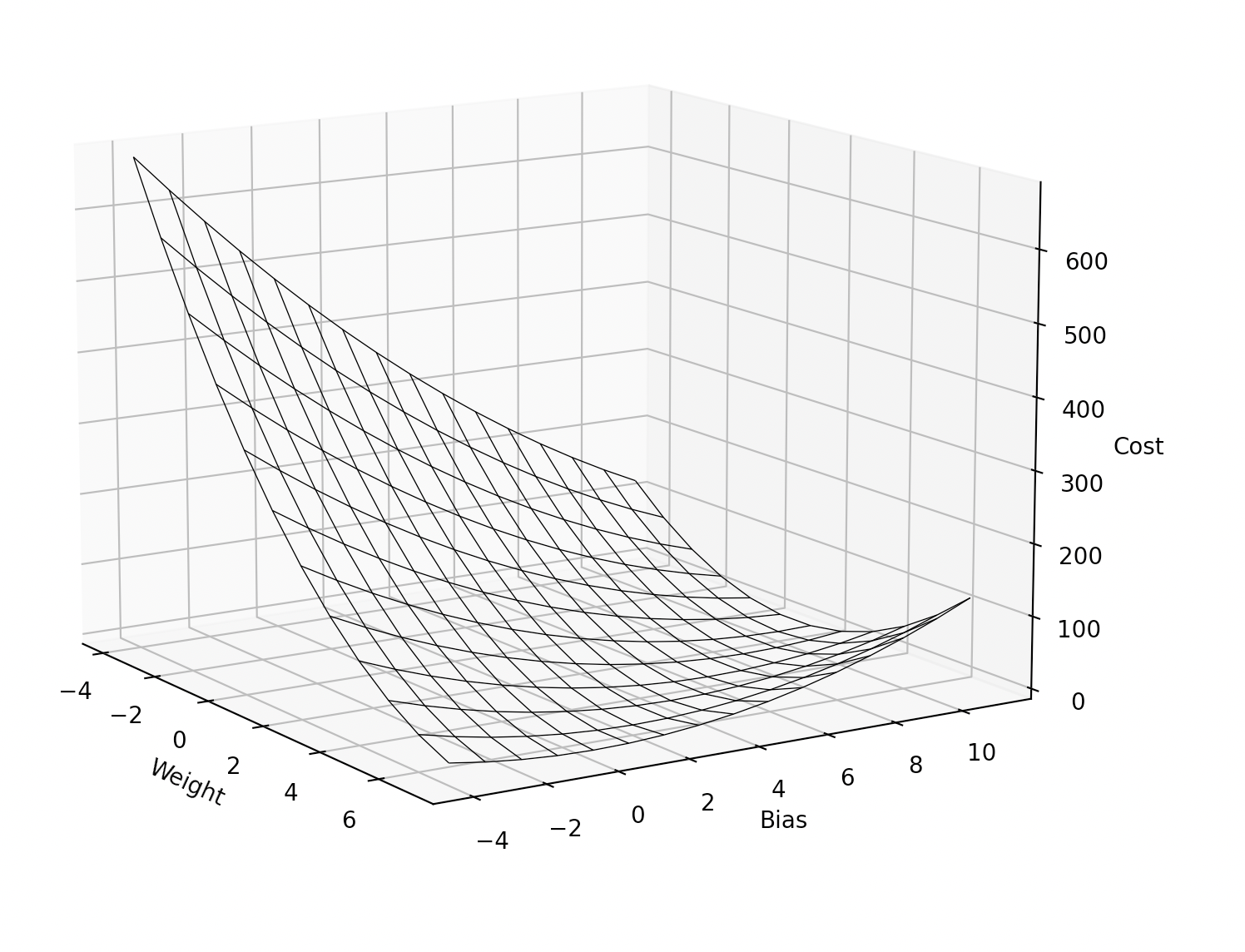This code shows how to create a diagram that visualises the relationship between a weight, bias and cost.

# Cost function diagram with matplotlib.

For my training "Create a neural network from scratch with Python", I needed to show a diagram that visualises the cost function.

The training data contains of a single input and single output:

```training_data_input = 2
training_data_output = 14  # input * 2 + 10```

As you can see, the weight should be 2 and the bias should be 10.

For this single training point, the cost needs to be calculated for a weight and bias within a certain range:

`weights, biases = np.meshgrid(range(-4, 8), range(-4, 12))`

## Cost function

Here is the cost function. It is the Mean Squared Error cost function:

`(training_data_output - (w * training_data_input + b)) ** 2`

The result:Here is the code to create the diagram:

```import matplotlib.pyplot as plt
import numpy as np

training_data_input = 2
training_data_output = 14  # 2 * 2 + 10

def cost(w, b):
return (training_data_output - (w * training_data_input + b)) ** 2

fig = plt.figure()
weights, biases = np.meshgrid(range(-4, 8), range(-4, 12))
costs = cost(weights, biases)
ax.plot_wireframe(weights, biases, costs, rstride=1, cstride=1, linewidth=0.5, edgecolor='black')

ax.set_xlabel('Weight')
ax.set_ylabel('Bias')
ax.set_zlabel('Cost')
plt.show()```

## Interesting Curve.

One of the things I noticed, is that the lowest cost is not a point, but many points on a line in the diagram.This is because many combinations of weight and bias can cause a low cost and that is one of the reasons why it is important to have a lot of training data while training a neural network!

Written by Loek van den Ouweland on 2021-11-16.
Questions regarding this artice? You can send them to the address below.